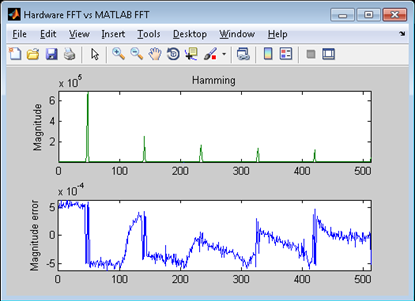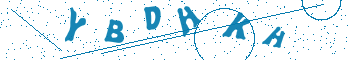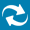# Controlling Riviera-PRO from MATLAB®

## Introduction

Riviera-PRO provides a bidirectional MATLAB co-simulation interface that allows the integration of the MathWorks' system-level computing environment with Aldec's HDL-based simulation environment. The interface enables engineers to verify that their ASIC/FPGA implementations match system specifications. Riviera-PRO can either act as a master or slave in co-simulation with MATLAB:

1. Master mode enables Riviera-PRO users to extend the testbench capabilities of HDL languages with the computing power of the MATLAB environment. This includes executing MATLAB® commands, calling M-functions, or transferring data to or from the MATLAB workspace. So, while remaining in the Riviera-PRO (HDL) environment, you can control the MATLAB environment. If you want to pursue this approach please refer to the following application note: www.aldec.com/support/resources/documentation/articles/1263

2. Slave mode enables MATLAB users to play with a bit-accurate model of their IP without even having to leave the MATLAB environment. Riviera-PRO’s MATLAB interface supports the flow in which you can keep MATLAB as a main environment and can call Riviera-PRO for HDL simulations. This way, you may not even need to deal with the HDL simulation environment at all (given your design-specific interface implemented by someone else at the HDL side). Please continue reading if you want to pursue this approach.

NOTE: In both approaches listed above, all data transfer operations over the interface are controlled from your HDL code. Communication with MATLAB is accomplished with a dedicated set of subprograms prepared for both Verilog and VHDL.

## Implementing Your Design-Specific Interface

You can skip to the next section if your HDL model already has the interface implemented. The MATLAB interface operation is based on calling interface functions within the HDL code. If you need to implement the interface from scratch, refer to the following application note: www.aldec.com/support/resources/documentation/articles/1263

### Interface highlights

• No limits on where the interface routines are inserted in the code – you can put them into the testbench or even IP code (allows pulling data out to MATLAB from any point of the HDL code).

• Built-in conversion of fixed-point data (HDL vectors) to MATLAB floating-point data (double, single) and integer types (HDL real to MATLAB double/single and HDL to MATLAB integer)

• Array transfers and efficient memory management

• HDL simulation time can be transferred to MATLAB

## Setting up MATLAB for Co-Simulation

In order to setup the interface, follow the configuration procedure described below:

1. Start MATLAB.

2. Change the current directory in the MATLAB window to the \$aldec/interfaces/matlab directory.

3. Type setup in the MATLAB Command Window and press Enter. The appearing warning dialog box prevents you from accidental removal of previous versions of the Riviera-PRO Toolbox installed in MATLAB. Press Yes to continue. The following message should be displayed in the Command Window:

```Welcome to Riviera-Pro Toolbox Setup.
Installing Riviera-Pro Toolbox...
Adding Riviera-Pro Toolbox path:
C:\Aldec\Riviera-PRO-2012.10-x64\interfaces\matlab
```

The Riviera-Pro Toolbox has been installed successfully. After the setup is finished, you are able to use the Riviera-PRO Toolbox immediately. The Riviera-PRO Toolbox comprises of the MATLAB and Simulink Interfaces.

## Running Simulation from MATLAB

Here are the basic steps you need to follow to run simulation from MATLAB:

1. Make sure you have implemented your design-specific interface

2. Start MATLAB and create an M-script in it

3. Execute (run) this script in MATLAB which will call Riviera-PRO and perform HDL simulation

Riviera-PRO uses commands to perform HDL simulation. Please refer to the manual at www.aldec.com/resources/manuals/Riviera-PRO/index.htm for more detail on how to use Riviera-PRO macro commands. Below is the sample script to perform HDL compilation and simulation from the MATLAB environment (based on the fft_analysis sample design available with Riviera-PRO installation at /examples/tools/matlab):

```%Initialize Riviera-PRO from Matlab
riv_server_init

%Compile project
riv_do('alib work')
riv_do('acom -dbg src/cfft/*.vhd')
riv_do('acom -dbg src/*.vhd')

%Execute 6 simulation for different window_type 'w' value
for w=1:6
asimstring = ['asim -ieee_nowarn -Gwindow_type=' num2str(w) ' top_fft_tb']
riv_do(asimstring)
riv_do('run -all')
riv_do('endsim')
%Collect all 6 results to the common arrays
all_fft_Qin(w,:)=fft_Qin
all_fft_Qout(w,:)=fft_Qout
end
```

Where,

• riv_server_init is a command to invoke Riviera-PRO from MATLAB.

• riv_do is a routine which will pass a string or command to Riviera-PRO for execution.

• -G argument enables you to pass different parameters to the HDL simulation initialization command (asim). The sample script runs simulation with 6 different window type functions used to perform simulation of the fft block in the same session.

Please use the above script from MATLAB (from fft_analysis directory) to perform co-simulation. Here are the results of the sample script execution:## Conclusion

Riviera-PRO can be started from MATLAB, and execute designs with MATLAB interface code. It allows creating robust testbench scenarios with extensive math data analysis of multiple HDL simulation runs within one MATLAB instance.

Ask Us a Question
x
Ask Us a Question
x
 Name: Phone: Email: Question: Security code:Incorrect data entered.
Thank you! Your question has been submitted. Please allow 1-3 business days for someone to respond to your question.